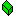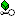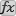e-CryptIt Engine Xojo Plugin

#SHA1 Class (console safe)

SHA1 is a message digest/hash algorithm with a digest/hash size of 160 bits (20 characters).

This algorithm has been tested against official test vectors.

Object
SHA1

class SHA1 implementsIHashAlgorithm

#### MethodsFinal (console safe) Use this function when you are done adding bytes to the SHA1 class.Update (console safe) Use this method to add data with MemoryBlock to the SHA1 stream.Update (console safe) Use this method to add data with String to the SHA1 stream.

#### Examples

Dim data as String
Dim hash as SHA1
Dim result as String
Dim hexResult as String
Dim i as Integer

data = "abc"

// We do this in ASCII because the well known test vectors come in ASCII
data = ConvertEncoding(data,Encodings.ASCII)

hash = new SHA1()

hash.Update(data)

result = hash.Final()

// Convert to HEX
For i = 1 to 20
hexResult = hexResult + Right("0"+Hex(Asc(Mid(result,i,1))),2)
next

if hexResult <> "A9993E364706816ABA3E25717850C26C9CD0D89D" then
return false
end if

// Lets test another official vector

data = "abcdbcdecdefdefgefghfghighijhijkijkljklmklmnlmnomnopnopq"

// We do this in ASCII because the well known test vectors come in ASCII
data = ConvertEncoding(data,Encodings.ASCII)

hash = new SHA1()

hash.Update(data)

result = hash.Final()

hexResult = ""
// Convert to HEX
For i = 1 to 20
hexResult = hexResult + Right("0"+Hex(Asc(Mid(result,i,1))),2)
next

if hexResult = "84983E441C3BD26EBAAE4AA1F95129E5E54670F1" then
return true
else
return false
end if

#### Supported Platforms:

• MacOS X Carbon
• MacOS X Cocoa 32 bit
• MacOS X Cocoa 64 bit
• Windows 32 bit
• Windows 64 bit
• Linux 32 bit
• Linux 64 bit
• Linux ARM